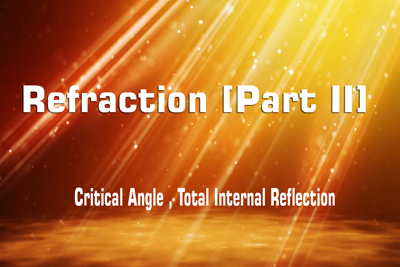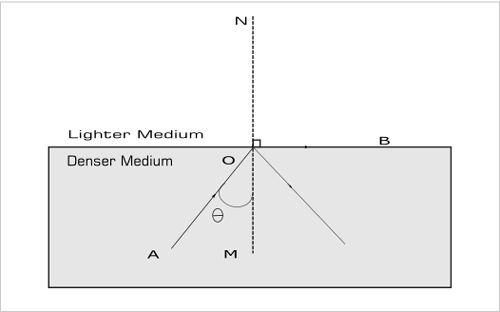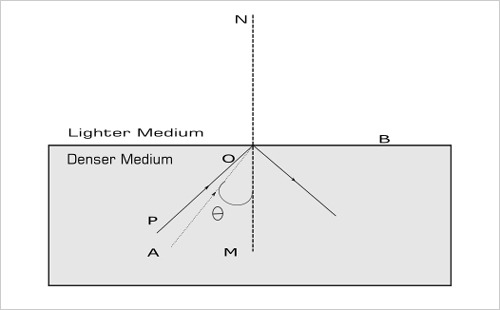Refraction - Critical Angle , Total Internal Reflection
27-01-2020    369 times### Laws of refraction

The refraction of light meets the following two law –

1. The normal at the point of incidence, the incident ray and the refracted ray are lies in the same plane.
2. The ratio of the sine of the angle of incidence (i) and the sine of the angle of refraction (r) are constant for the pair of given media. This constant is called the refractive index of the second medium with respect to the first medium. It is generally represented by the Greek letter mew (1&mew;2) or 1n2. This law is also known as snell’s law.

(sin i /sin r) = 1μ2

The refractive index has no unit as it is the ratio of two similar quantities.

### Critical angleWhen a light ray refracted from a denser medium to lighter medium, then the incidence angle of a denser medium reaches a certain critical value, such that the refracted angle will be 90&deg;, that is refracted ray lies along the boundary. This certain value of the incidence angle is known as the critical angle of those mediums.

If light ray meets the following conditions then a critical angle can produce

1. Light ray must travel to the lighter medium from a denser medium. And refracted from the boundary of the two different mediums.
2. The refracted angle of the lighter medium must be 90&deg;

The critical angle depends on the color of the light ray and the pair of the mediums. The critical angle of the red ray is greater than the green or a violet rays for a constant pair of the medium.

### Total internal reflectionWhen a light ray refracted from a denser medium to lighter medium and incidence angle is greater than critical angles (i>&theta;), then the refracted angle will be greater than 90&deg; and the refracted ray will return back to the denser medium. This phenomenon is known as total internal refraction.

If light ray meets the following conditions then total internal reflection can produce

1. Light ray must travel to the lighter medium from a denser medium. And refracted from the boundary of the two different mediums.
2. The incidence angle of the denser medium is greater than the critical angle of that medium.